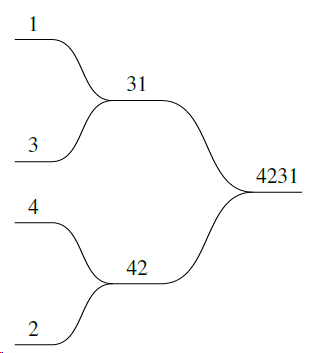## Fusing Numbers

Two numbers can be fused by putting one in front of the other to make the largest number possible. For example, 29 and 71 can be fused to form 7129.

A list of numbers can be fused by repeatedly fusing pairs of numbers of the same length to form the biggest number possible.

For example, given the sequence 1 3 4 2, the 1 and 3 are fused to give 31 and the 4 and 2 are fused to give 42. Then the 31 and 42 are fused to give 4231.Question:

If the original sequence is 4 7 5 6 8 2 1 3, what is the last digit of the final fused number?